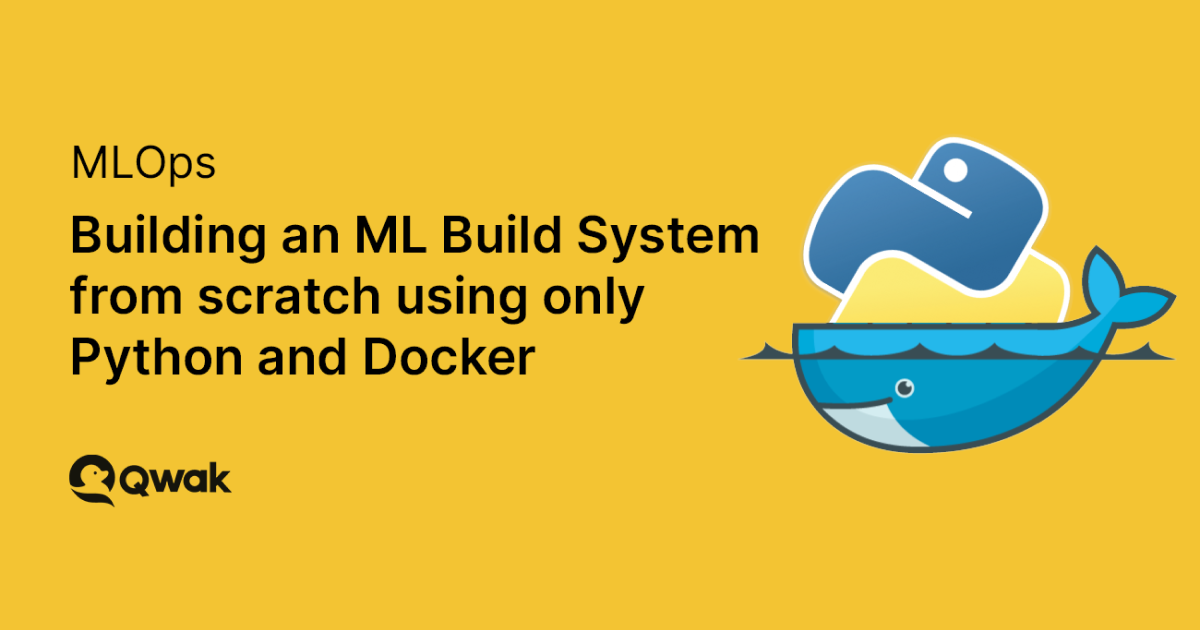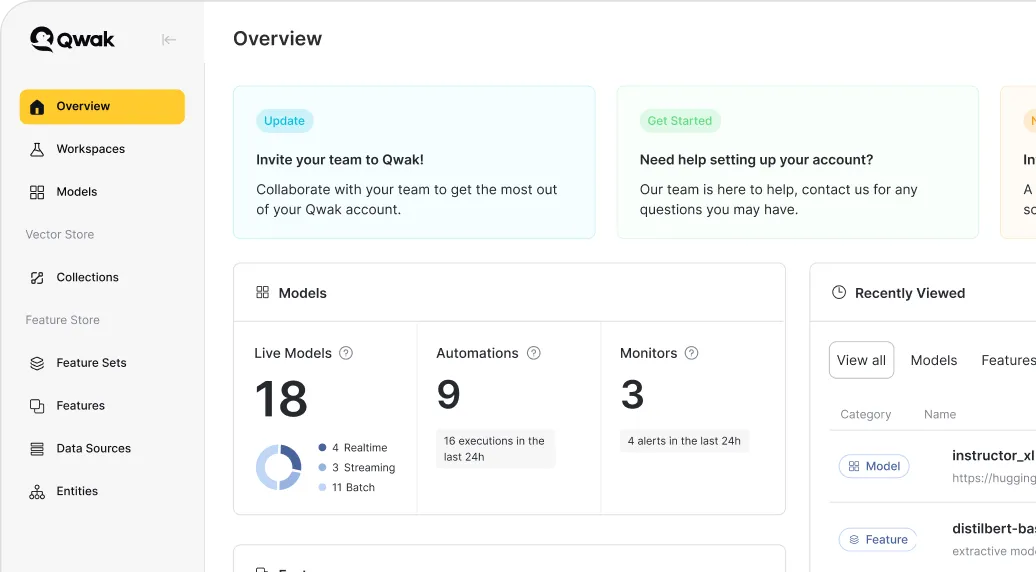# Building an ML Build System from scratch using only Python and Docker

In this article, we will show you how to implement a build system that retrieves the training data, trains the model, and creates a Docker container with a REST service generating the model predictions. Our model will perform a well-known task of predicting whether a Titanic passenger survives or dies.Previously, we described why every MLOps team needs a proper ML Engineering platform. We defined the challenge of building an integrated ML system and running the models in production. We discussed model reproducibility, version control, testing, and model serialization. It's time to get all of that into production!

In this article, we will show you how to implement a build system that retrieves the training data, trains the model, and creates a Docker container with a REST service generating the model predictions. Our model will perform a well-known task of predicting whether a Titanic passenger survives or dies.

In this example, we will not use any orchestration services, MLOps platforms, or tools facilitating model packaging and deployment. Instead, we will implement everything step-by-step in pure Python code.

## How to Run the Build Script

A build system is a part of a puzzle that consists of multiple components such as data processing, model building, model deployments, etc. In production use cases, we orchestrate the process using tools like Airflow, MetaFlow, and Perfect. In this blog, however, we focus on the build phase. Therefore, we will not use an orchestration service.

Before we start, we have to specify the dependencies. In this example, we'll use Pipenv to configure a virtual environment. We will need three dependencies:

• pandas: we'll use the library to preprocess the data and, later, to select the relevant value from the model inference.
• scikit-learn:  we need it to split the dataset into the training and the test set.
• CatBoost:  we use it to train the classifier model, perform cross-validation, and download the input data.

In the Pipfile, we have the following values:

``````[[source]]

url = "https://pypi.org/simple"
verify_ssl = true
name = "pypi"

[packages]

[dev-packages]
catboost = "*"
pandas = "*"
scikit-learn = "*"

[requires]
python_version = "3.9"
``````

Now, we can create a new virtual environment and download the dependencies:

``````pipenv install --dev
``````

## Getting the Input Data

Data changes in a production environment, and we'll retrieve the training data from the organization data lake or a feature store. However, our example model uses a constant dataset, and we won't need to retrain it ever again, so we’ll use the CatBoost library to download the dataset from its examples repository.

We put the following code into the data_preprocessing.py file:

``````import pandas as pd
from catboost.datasets import titanic
if __name__ == '__main__':
titanic_train, _ = titanic()
``````

In the same file, we will also deal with missing values and remove the useless columns.

First, we replace nulls with a number that can be easily distinguished (and ignored) by the trained model:

``````titanic_train.fillna(-999, inplace=True)
``````

After that, we remove the PassengerId column:

``````preprocessed = titanic_train.drop(['PassengerId'], axis=1)
``````

In our simple example, we don't need more data preprocessing, so now we can store the preprocessed data in a CSV file:

``````preprocessed.to_csv('preprocessed_data.csv', index=False)
``````

## Training the Model and Serialization

The next part of the build script will train an ML model and store it in a file.

In the beginning, we must load the preprocessed data and split it into independent features and the target variable:

``````import pandas as pd
import numpy as np
from catboost import CatBoostClassifier, Pool, cv
from sklearn.model_selection import train_test_split

if __name__ == '__main__':

x = data.drop(['Survived'], axis=1)
y = data.Survived
``````

Now, we can configure the CatBoostClassifier classifier.

``````loss_function= 'Logloss'
learning_rate= None
iterations= 1000
custom_loss='Accuracy'

model = CatBoostClassifier(iterations=iterations,
custom_loss=[custom_loss],
loss_function=loss_function,
learning_rate=learning_rate
``````

After model configuration, we do the final data preparation. We need to split the dataset into training and test sets. We also need to pass a Boolean vector indicating which features are categorical variables; CatBoost will deal with them automatically:

``````x_train, x_test, y_train, y_test = train_test_split(x, y, train_size=.85, random_state=42)
is_categorical_feature = np.where(x_train.dtypes != float)

``````

Finally, we can train the model using five-fold cross-validation. In the last step, we save the trained model to a file:

``````model.fit(x_train, y_train, cat_features=is_categorical_feature, eval_set=(x_test, y_test))
cv(Pool(x, y, cat_features=is_categorical_feature), model.get_params(), fold_count=5)

model.save_model('trained_model.cbm')
``````

We have trained the model and saved it in a file. Is this enough? Are we done?

Unfortunately, a trained model is not a deployable artifact yet. If we sent the model to another person, they could not use it to generate predictions. What is missing?

Besides the model, the deployable artifact contains all the dependencies required for the inference. In this example, we need the correct version of CatBoost, scikit-learn, and pandas.

Why do we need pandas? CatBoost expects the input in a pandas DataFrame containing the preprocessed data. Preprocessed data! We need to copy some of the preprocessing code to the artifact. Otherwise, we can’t use the model. In our example, the preprocessing part was relatively easy—we removed the PassengerId column. Let’s look at how we can turn the saved model into a deployable artifact using Docker and Flask.

## Serving the Model

We want to deploy the model as a REST service. Therefore, we will need a web server. Our web server uses the Flask library, loads the model from a file, and exposes a POST HTTP endpoint to handle the requests:

``````from flask import Flask, request, jsonify
import pandas as pd
from catboost import CatBoostClassifier

model = CatBoostClassifier()

@app.route('/predict', methods=['POST'])
def predict():
content = request.json
df = pd.DataFrame(content, index=)
df = df.drop(['PassengerId'], axis=1)
print(df)
result = model.predict_proba(df)
result = {'Survived_Probability': result[0, 1]}
return jsonify(result)

if __name__ == '__main__':
app.run(debug=True,host='0.0.0.0')
``````

Note that we loaded the model in the code outside the predict function! We don't want to load it during every request.

In the predict function, we get a POST request containing a JSON body. The content gets parsed as a pandas DataFrame, and we remove the redundant PassengerId column. When the data is ready to use, we pass it to the model's predict_proba function. The function returns an array of two values, but we care only about the survival probability, which we extract from the result.

## Building the Endpoint

We are not done yet. In the next step, we’ll build a Docker image containing the entire service.

For the build, we need a Dockerfile containing the base image (python:3), the required dependencies, the model, and the service code:

``````FROM python:3
RUN pip install --no-cache-dir flask catboost pandas scikit-learn

COPY service.py /app/service.py
COPY trained_model.cbm /app/trained_model.cbm

WORKDIR /app
EXPOSE 5000
ENTRYPOINT ["python"]
CMD ["service.py"]
``````

Finally, we can run the docker build command to get the image:

``````docker build -t mlmodel:latest .
``````

## Testing the Endpoint

An MLOps platform is not complete if it lacks the testing feature. Therefore, we'll now start the Docker container and send a test request to check whether we get the expected response.

``````docker run -p 5000:5000 mlmodel:latest &
``````

The best way to test the service is to prepare test cases and write a Python script to send the request to the locally running Docker container. However, even that can be simplified  by using curl, diff, and tr command-line tools to test the model:

``````diff <(curl -d '{"PassengerId": 762, "Pclass":3, "Name": "Nirva, Mr. Lisakki Antino Aijo", "Sex": "female", "Age": 34, "SibSp": 4, "Parch": 3, "Ticket": "a", "Fare": 1.0, "Cabin": "A", "Embarked": "A"}' -H 'Content-Type: application/json' http://localhost:5000/predict  | tr -d "\n ") <(echo '{"Survived_Probability":0.6485056503550757}' | tr -d "\n")
``````

## What to do Next

Now you can deploy the model! Because we built a Docker image, you can choose any deployment service you want—for example, a Kubernetes cluster or any tool that can run a Docker container.

Before deploying, however, you must upload the Docker image into a Docker registry. In this tutorial, we will use the Amazon Elastic Container Registry:

``````docker build --tag aws_account_id.dkr.ecr.aws_region.amazonaws.com/model_name:version .
\$(aws ecr get-login region aws_region --no-include-email)
docker push aws_account_id.dkr.ecr.aws_region.amazonaws.com/model_name:version
``````

Of course, the solution described in this text is model-specific. If we want to deploy a different model, we must change every step in the build script. It may be a good enough approach when you have one or two models, but if you run multiple models in production, then it would be great if they shared at least some of the build code. Imagine the horror of upgrading libraries when every build script is different.

What if we want to deploy multiple models in one service? For example, to run an A/B test of different model versions. Would it be possible to do it using our build script? For sure, but we would have to duplicate almost all of the code, add the implementation to assign requests to a model in the web server randomly, and modify the response to tell the user which version generated the prediction.

Would this implementation handle a large number of requests? Flask is a highly scalable web framework, but when running an ML model, it may slow down. After all, an ML model may require a few GBs of memory to store the parameters. Such huge models are powerful but not fast. We would need to deploy multiple service instances to keep up with the requests.

In the next blog post, we'll show you how to use Qwak to train a model, build a deployable artifact, test it, and deploy it.
We will need to write less than half of the code shown in this article. For Qwak, we need only the training code, inference preprocessing, and test cases. Qwak handles everything else automatically, so we don't need to worry about it.

Chat with us to see the platform live and discover how we can help simplify your ML journey.